Mobile QR Code1. (Electrical Engineering, Pohang University of Science and Technology, 77, Cheongam-ro, Nam-gu, Pohang-si, Gyeongsangbuk-do, Pohang 37673, Korea )

## I. INTRODUCTION

Successive approximation register (SAR) ADC has been the most preferred candidate in low-power sensor applications (1-5). Though it can provide a burst-mode conversion for the duty-cycled system with superior energy efficiency, achievable effective number of bits (ENOBs) are limited to 10~11b. For sensor applications requiring an ENOB of over 14b, oversampling ADCs using delta-sigma modulators (DSMs) (7-10) have been general candidates, but at the cost of more energy consumption. To mitigate the problem of comparator noise in SAR ADC, two-step pipe-lined-SAR architecture with a gain stage between two SAR steps has been actively investigated (6). However, the mismatch among capacitors and tightened requirement of high-precision analog circuits for inter-stage processing eventually limit the maximum achievable ENOBs.

Residue-integration (RI) SAR ADCs (11-13) have been also considered for fine-precision applications. Though they demonstrated effectiveness both in robustness against comparator noise and in energy efficiency, they require a high-gain amplifier for RI (11,12). In (13), combining a DSM in RI operation helps to relieve the constraint on the amplifier gain. Though it reduces the requirement of high-precision analog circuits, mismatch among capacitors is still left to be the performance-limiting factor. Adopting dynamic element matching (DEM) can reduce the effect of capacitor mismatch. However, applying DEM brings about complicated circuit blocks and is limited to only a part of capacitor bank of SAR ADC (13,14).

The calibration technique has been considered to compensate for capacitor mismatches, hence improving the code linearity (15-20). The effect of capacitor mismatch could be also reduced by employing a redundancy (15), but at the cost of complicated processing of sub-2 radix-based conversion. As an alternative approach, background calibration schemes (16-20) have been actively investigated. However, they cause complicated digital logic and require a long time with a wide-range of input to collect sufficient statistical information for the calibration.

This paper presents a digital-domain capacitor mismatch calibration scheme in RI SAR ADC. For verification, a 16-bit ADC is implemented in 180 nm CMOS technology, achieving an SNDR of 87.50-dB, an ENOB of 14.24b, and an SFDR of 106.85-dB. Section 2 describes the circuit and algorithm for the calibration. Section 3 shows measurement results, and Section 4 concludes this work.

Fig. 1. Architecture of the proposed SAR ADC in (a) conversion mode, (b) calibration mode.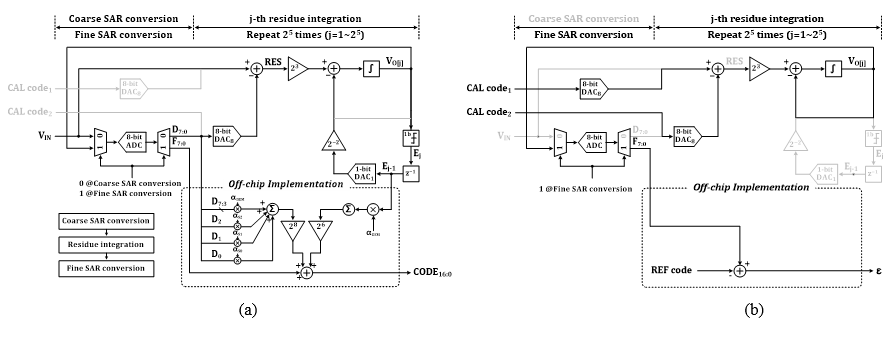Fig. 2. Circuit configurations in the conversion mode for (a) coarse/find SAR conversion, (b) residue integration.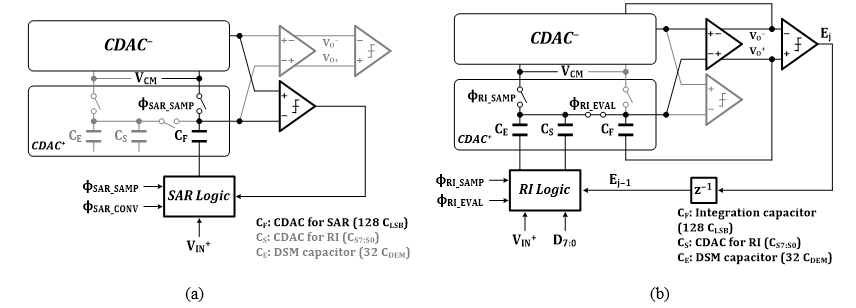## II. Circuit Description

### 1. Architecture

The designed ADC is based on (13), operating with two modes, conversion mode and calibration mode (Fig. 1). In the conversion mode, ADC receives the analog input V$_{\mathrm{IN}}$ and quickly performs 8-bit asynchronous SAR conversion, generating coarse 8 MSBs. Then, the residue integration is performed while receiving V$_{\mathrm{IN}}$. It accumulates the quantization error by estimating V$_{\mathrm{IN}}$ with the coarse 8 MSBs. After repeating the integration 2$^{5}$ times, the result is quickly converted again with the same SAR conversion used in the first step, generating fine 8 bits. During the residue integration step, a DSM loop simultaneously converts 1-bit output (E$_{\mathrm{j}}$) in parallel at each of 2$^{5}$ integration cycles. It helps to confine the amount of integrated residue and greatly relieves the requirement of amplifier gain. The total sum of two SAR conversion outputs and DSM outputs becomes the final digital output. In the summing process, the 8-bit output of the first SAR conversion are scaled up by 2$^{8}$. Since each DSM output affects the residue with a significance of the 5$^{\mathrm{th}}$ bit and the residue is then amplified by 2$^{3}$, the DSM output should be scaled by 2$^{8{-}5+3}$, or 2$^{6}$. Detailed circuit operation in the conversion mode is explained in Fig. 2. A DEM is applied to 5 MSB capacitors for averaging. The capacitors for 5 MSBs (C$_{\mathrm{S7:S3}}$) are implemented in a segmented capacitor array with each capacitance of 32C$_{\mathrm{DEM}}$. The 31 capacitors of C$_{\mathrm{S7:S3}}$ and a DSM capacitor (C$_{\mathrm{E}}$) form 32 identical capacitors for DEM. The DEM rotates the role of each capacitor during one full conversion of 32 cycles.

Fig. 3. Simulated INL with amplifier gain of (a) 1024, (b) 512, (c) 256.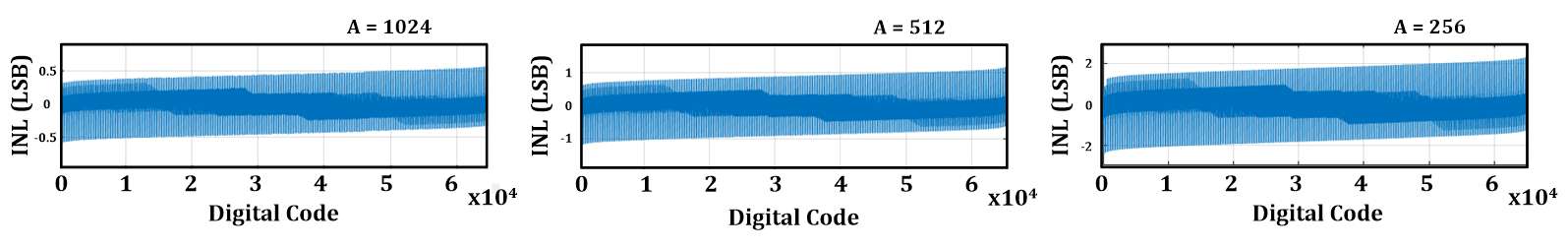The RI with DSM greatly relieves the required open-loop amplifier gain. Assuming that V$_{\mathrm{DD}}$ is the input conversion range, the maximum amplifier output (V$_{\mathrm{o,max}}$) during RI process confined by DSM operation becomes

##### (1)
$\left|V_{o, \max }\right| \leq 2^{-5+3} \cdot V_{D D}=2^{-2} \cdot V_{D D}$

Therefore, the maximum nonzero difference at the amplifier input during RI process due to the finite amplifier gain, A, is

##### (2)
$\frac{2^{-2} \cdot V_{D D}}{A}$

which becomes the input-referred error at the amplifier input. Since the inter-stage closed-loop gain for the fine conversion can be reduced by the number of RI cycle (2$^{5}$), the maximum error at the amplifier output for the fine conversion is calculated to be

##### (3)
$\frac{2^{8}}{2^{5}}\frac{2^{- 2}\cdot V_{DD}}{A}$

which should be less than 1 LSB of the 8-bit fine conversion, or $V_{DD}/2^{8}$. It leads to the requirement for A, as

##### (4)
$A>512\left(=54.3\,\,\mathrm{dB}\right)$

Thus, the requirement for the amplifier gain is effectively reduced with factors of 2$^{-2}$ and 2$^{-5}$ by DSM and RI, respectively. Fig. 3 shows simulated INL for different amplifier gains, revealing the validity of the analysis.

In the calibration mode, a test input is self-generated by applying a known digital code. For the estimation of capacitor mismatch, two different combinations of digital codes representing the same amount of test input are prepared. Once the two digital codes are given, the residue integration is directly processed without the coarse conversion step. While one digital code provides an equivalent input, the other digital code performs the role of the coarse MSBs. The result of residue integrations is supposed to be 0 if the capacitors are perfectly matched. A nonzero residue is then converted through the subsequent fine SAR operation. Thus, the amount of capacitor mismatch among the sampling capacitors can be extracted from the ADC output code.

### 2. Calibration Algorithm

The calibration mode estimates the effect of mismatches of the eight MSB capacitors used for the input sampling during residue integration. The error is represented in a form of a ratio with the feedback capacitance ($128C_{LSB}$). Fig. 4 shows how to estimate the capacitance mismatch error. Let the dummy capacitor ($C_{DUMMY}$) of $4C_{LSB}$ be the reference. The capacitances, $C_{LSB}$, $C_{S0}$, $C_{S1}$, $C_{S2}$ and $C_{DEM}$ are identical if there is no error. To measure each capacitor error, two given calibration codes (CAL code$_{1}$, CAL code$_{2}$) are applied as the input and the estimated code for residue integration, respectively. The mismatch between $4C_{S0}$ and $C_{DUMMY}$ leads to a nonzero residue at the amplifier output after the first cycle of the residue integration, which is

##### (5)
\begin{align} \begin{array}{l} V_{O\left[1\right]}=V_{O\left[1\right]}^{+}- V_{O\left[1\right]}^{- }\\ =\left(\frac{4C_{S0}}{128C_{LSB}}\left(V_{REF}- 0\right)+\frac{4C_{LSB}}{128C_{LSB}}\left(0- V_{REF}\right)\right)\\ - \left(\frac{4C_{S0}}{128C_{LSB}}\left(0- V_{REF}\right)+\frac{4C_{LSB}}{128C_{LSB}}\left(V_{REF}- 0\right)\right)\\ =\frac{1}{16}\frac{C_{S0}}{C_{LSB}}V_{REF}- \frac{1}{16}V_{REF} \end{array} \end{align}

After the 32nd integration, the output becomes

##### (6)
$V_{o\left[32\right]}=32V_{o\left[1\right]}=32\times \left(\frac{1}{16}\alpha _{S0}- \frac{1}{16}\right)V_{REF}$

where $\alpha _{S0}=C_{S0}/C_{LSB}$. The $\alpha _{S0}$ becomes 1, or $V_{o}$ is 0, if there is no mismatch error. Subsequent processing of $V_{o}$ by the fine AD conversion reveals the information of mismatch error in a form of digital code. Defining $\varepsilon _{S0}$ to be the mismatch error in the capacitor $C_{S0}$,

##### (7)
$\alpha _{S0}=1+\varepsilon _{S0}$

Fig. 4. (a) Circuit configurations, (b) code sets for estimation of capacitor mismatch in the calibration mode.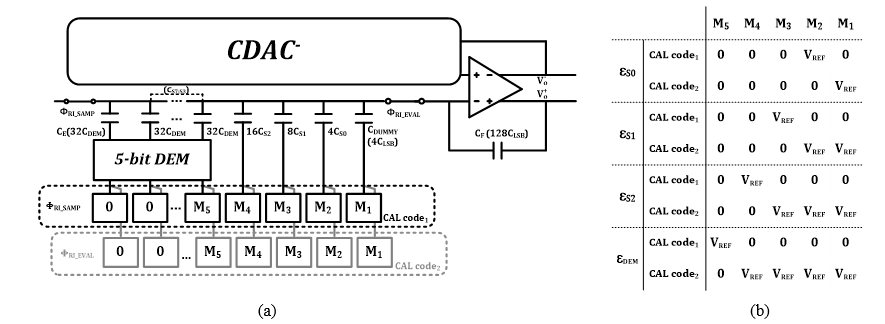The mismatch error for $C_{S1}$, represented by $\varepsilon _{S1}$, can be also derived in a similar way using the previously obtained $\varepsilon _{S0}$. Since the five MSB capacitors (C$_{\mathrm{S7:S3}}$) and C$_{\mathrm{E}}$ are averaged by DEM, the mismatch error for those capacitors can be represented by a single parameter with an averaged error. The capacitance ratios can be obtained as follows:

##### (8)
\begin{align} \begin{array}{l} \alpha _{S1}=\frac{1}{2}+\frac{1}{2}\alpha _{S0}+\varepsilon _{S1}\\ \alpha _{S2}=\frac{1}{4}+\frac{1}{2}\alpha _{S1}+\frac{1}{4}\alpha _{S0}+\varepsilon _{S2}\\ \alpha _{DEM}=\frac{1}{8}+\frac{1}{2}\alpha _{S2}+\frac{1}{4}\alpha _{S1}+\frac{1}{8}\alpha _{S0}+\varepsilon _{DEM} \end{array} \end{align}

where $\alpha _{DEM}=C_{DEM}/C_{LSB}$, $\alpha _{S2}=C_{S2}/C_{LSB}$ and $\alpha _{S1}=C_{S1}/C_{LSB}$. The mismatch errors for capacitors C$_{\mathrm{S1}}$, C$_{\mathrm{S2}}$ and C$_{\mathrm{S7:S3}}$ are represented by $\varepsilon _{S1}$, $\varepsilon _{S2}$ and $\varepsilon _{DEM}$, respectively. The digitally estimated capacitance ratios ($\alpha _{DEM}$, $\alpha _{S2}$, $\alpha _{S1}$ and $\alpha _{S0}$) are used in the conversion mode by multiplying $\alpha _{DEM}$ to D$_{\mathrm{7:3}}$, $\alpha _{S2}$ to D$_{2}$, $\alpha _{S1}$ to D$_{1}$, $\alpha _{S0}$ to D$_{0}$ and $\alpha _{DEM}$ to E$_{\mathrm{31:0}}$, respectively. These multiplications can be simply implemented by addition of binary-weighted digitized errors ($\varepsilon _{S0}$, $\varepsilon _{S1}$, $\varepsilon _{S2}$ and $\varepsilon _{DEM}$).

To verify the calibration algorithm, we applied random mismatch to DAC which is comprised of unit capacitors. Fig. 5. shows simulated results when Gaussian statistics are applied to MSB capacitors with standard deviations of 0.02 and 0.05. The calibration noticeably improves linearity performance without any missing code. The conventional calibration schemes by only manipulating the final output code without affecting capacitor DAC could result in missing codes. However, the proposed calibration is applied to the coarse 8 bits and DSM bits before the summation with the fine 8 bits. Since the use of DSM provides a nonzero overlap between coarse 8 bits, there is no missing code in the calibrated output.

Fig. 5. Simulation results according to MSB capacitor mismatch applied to Gaussian statistics with standard deviation of (a) 0.02, (b) 0.05.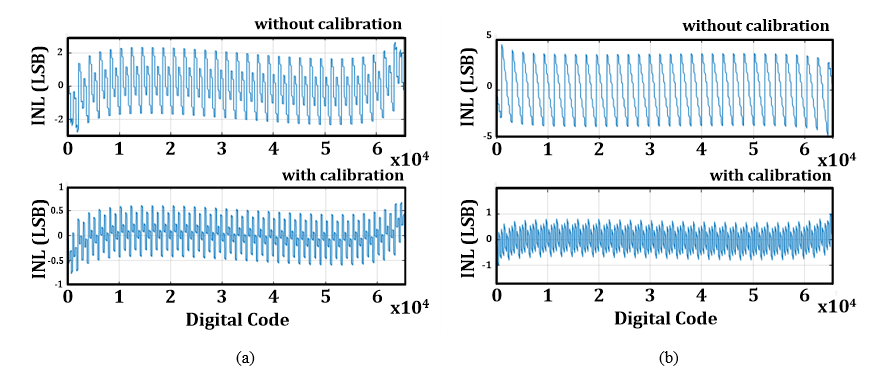## III. Measurement

The proposed ADC is fabricated using 180-nm CMOS technology, occupying a die area of 0.90 mm x 0.75 mm (0.68 mm$^{2}$). Fig. 6. shows a die photo. The unit capacitance is 20.28fF which is the smallest metal-insulator-metal (MIM) capacitor with the given technology. Using V$_{\mathrm{DD}}$ as V$_{\mathrm{REF}}$, a full-scale differential input signal of 3.6V$_{\mathrm{pp}}$ is converted. The chip provides the outputs of the two SAR conversions and the DSM codes to off-chip program, so that the calibration is verified through software.

Fig. 7 shows fast Fourier transform (FFT) spectra with a low-frequency input. Without the calibration, SNDR performance is mainly limited by harmonics caused by capacitor mismatches. However, the calibration improves SNDR by 5.9 dB and SFDR by 14.3 dB, achieving 87.5 dB and 106.85 dB, respectively. It represents an ENOB of 14.24-bit. A near Nyquist-rate input shows an insignificant degradation of performance (Fig. 8). The effect of calibration is also seen in code linearity (Fig. 9). There are outstanding repeated 32 patterns in INL and DNL plots before calibration. It is due to capacitor mismatch between averaged DEM capacitance and other MSB capacitances. While the peak INL lies within +3.56/-3.36 without the calibration, it is improved to +2.14/-2.08 with the calibration. In the whole range of the input frequency, SNDR and SFDR are improved by calibration (Fig. 10). Table 1 summarizes and compares performance with previously reported SAR ADCs whose resolution is higher than 12-bits. Since the final code is generated through software-based calibration, the total power consumption including digital-domain code scaling can be only expected by estimation. This work is continued research of (13) using the same core blocks. Additional power consumption for digital-domain code scaling at the output stage is estimated to be still negligible.

## IV. CONCLUSIONS

This work proposes a capacitor mismatch calibration scheme for a residue-integrated SAR ADC. Unlike the conventional DSM-driven oversampling noise-shaping

Fig. 7. The 65536-point FFT spectra calculated from measurement at a low-frequency input (a) without calibration, (b) with calibration.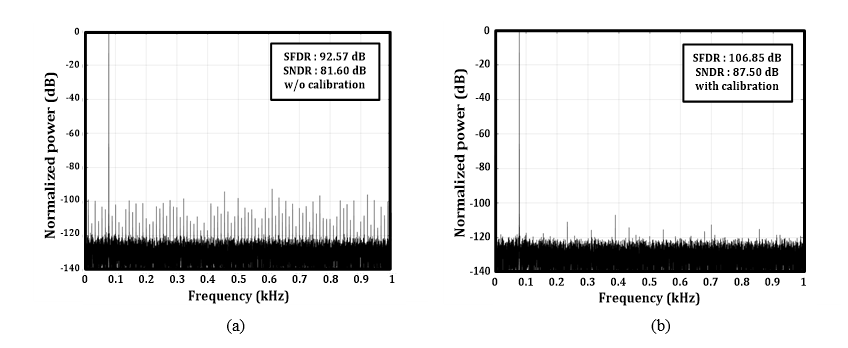Fig. 8. The 65536-point FFT spectra calculated from measurements at a near Nyquist-rate input (a) without calibration, (b) with calibration.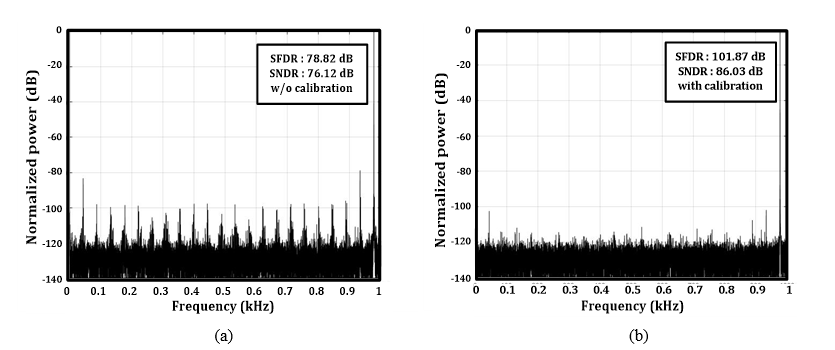Fig. 9. Measured code linearity (a) without calibration, (b) with calibration at sampling rate 2 kS/s.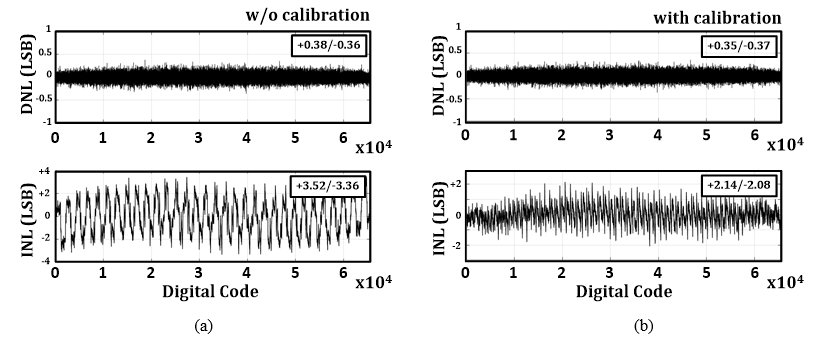ADC, the proposed ADC does not require any extra post-processing for decimation or filtering while performing integration of residue for noise averaging. Matching error in the binary-weighted input-sampling capacitances is estimated in a form of a digital number which is used for the scaling of corresponding digital number which is used for the scaling of corresponding digital code at the output stage during conversion. A 16-bit ADC is implemented using 180-nm CMOS technology. The calibration achieves 87.5 dB SNDR and 106.85 dB SFDR, showing improvements of 5.9 dB and 14.3 dB respectively.

Fig. 10. SNDR and SFDR vs. input frequency according to use of calibration at sampling rate 2 kS/s.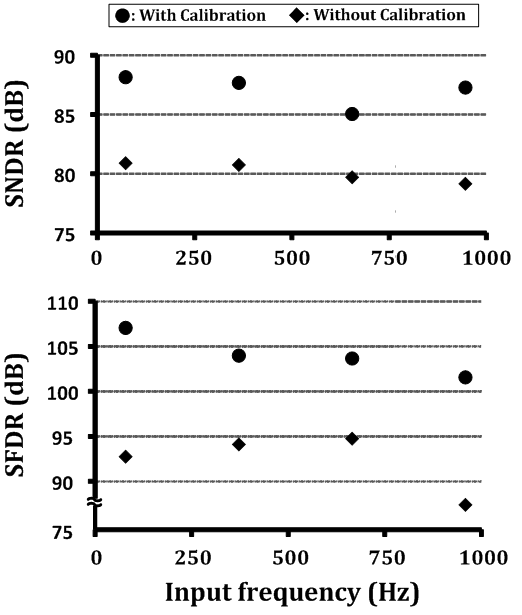Table 1. Performance Comparison with Nyquist-Rate ADCs

 This work JSSC’ 20 (22) IEEE’ 19 (21) TCAS’ 19 (5) JSSC’ 18 (13) VLSI’ 17 (17) ISSCC’ 17 (7) VLSI’ 16 (9) Architecture SAR SAR SAR SAR SAR SAR pipe. SAR SAR Technology [nm] 180 40 180 130 180 55 40 40 Resolution [bits] 16 13 16 12 16 16 14 15 Area [mm2] 0.68 0.005 5.61 0.16 0.68 0.55 0.342 0.32 fs [kS/s] 2 40k 1000 1 2 16k 35k 20 Power Supply [V] 1.8 1.1 5 1 1.8 5/2.5 - - Power [μW] 8.1* 591 40k 0.11 7.93 10.5k 21.8k 1.17 SFDRNyquist [dB] 106.85 79.2 107.9 78.5 98.2 82 94.3 95.1 SNDRNyquist [dB] 87.5 69 92.3 64.8 84.6 80** 75.1 74.1 FOMs [dB] 168.4* 174.3 166.3 161.4 165.6 165** 164.1 173.4

* : expected

** : with low frequency input

FOMs = SNDR + 10${\cdot}$log(BW/Power)

### ACKNOWLEDGMENTS

This work was supported by Engineering Research Center Program of the National Research Foundation funded by the Korea Ministry of Science and ICT (No. 2019R1A5A1027055).

### REFERENCES

1
Verma N., Chandrakasan A. P., Jun 2007, An ultra low energy 12-bit rate-resolution scalable SAR ADC for wireless sensor nodes, IEEE J Solid-State Circuits, Vol. 42, No. 6, pp. 1196-12052
Sharma A., Polley A., Lee S. B., Narayanan S., Li W., Sculley T., Ramaswamy S., Apr 2017, A Sub-60-μA multimodal smart biosensing SoC with > 80-dB SNR, 35-μA photoplethysmography signal chain, IEEE J Solid-State Circuits, Vol. 52, No. 4, pp. 1021-10333
Harpe P. J. A., Gao H., Dommele R. V., Cantatore E., van Roermund A.H. M., Jan 2016, A 0.20mm2 3nW signal acquisition IC for miniature sensor nodes in 65 nm CMOS, IEEE J Solid-State Circuits, Vol. 51, No. 1, pp. 240-2484
Zhu Z., Liang Y., Sep 2015, A 0.6-V 38-nW 9.4-ENOB 20-KS/s SAR ADC in 0.18-μm CMOS for medical implant devices, IEEE Trans Circuits Syst I Reg. Papers, Vol. 62, No. 9, pp. 2167-21765
Mao W., Li Y., Heng C.-H., Lian Y., Feb 2019, A low-power 12-bit 1-kS/s SAR ADC for biomedical signal processing, IEEE Trans Circuits Syst I Reg Papers, Vol. 66, No. 2, pp. 477-4876
Lee C. C., Flynn M. P., Apr 2011, A SAR-assisted two-stage pipeline ADC, IEEE J Solid-State Circuits, Vol. 46, No. 4, pp. 859-8697
Xu H., Cai Y., Du L., Zhou Y., Xu B., Gong D., Ye J., Chiu Y., Feb 2017, A 78.5dB-SNDR radiation- and metastability-tolerant two-step split SAR ADC operating up to 75MS/s with 24.9mW power consumption in 65nm CMOS, in Proc IEEE Int Solid-State Circuits Conf (ISSCC) Dig Tech Papers San Francisco CA USA, pp. 476-4778
Maddox M., Chen B., Coln M., Kapusta R., Shen J., Fernando L., Nov 2016, A 16 bit linear passive-charge-sharing SAR ADC in 55 nm CMOS, in Proc IEEE Asian Solid-State Circuits Conf (A-SSCC), pp. 153-1569
Shim M., Jun 2016, An oscillator collapse-based comparator with application in a 74.1 dB SNDR, 20 KS/s 15 b SAR ADC, in Symp VLSI Circuits Dig Tech Papers Honolulu HI USA, pp. 1-210
Harpe P., Cantatore E., van Roermund A., Feb 2014, An oversampled 12/14b SAR ADC with noise reduction and linearity enhancements achieving up to 79.1dB SNDR, in IEEE Int Solid-State Circuits Conf (ISSCC) Dig. Tech. Papers, pp. 194-19511
Hurrel C. P., Lyden C., Laing D., Dec 2010, An 18 b 12.5MS/s ADC with 93dB SNR, IEEE J. Solid-State Circuits}, Vol. 45, No. 12, pp. 2647-265412
Bannon A., Hurrell C. P., Hummerston D., Lyden and C., Jun 2014, An 18 b 5 MS/s SAR ADC with 100.2 dB dynamic range, in Symp VLSI Circuits Dig Tech Papers Honolulu HI USA, pp. 42-4313
Choi S., Ku H.-S., Son H., Kim B., Park H.-J., Sim J.-Y., Feb 2018, An 84.6-dB-SNDR and 98.2-dB-SFDR residue-integrated SAR ADC for low-power sensor applications, IEEE J Solid-State Circuits, Vol. 53, No. 2, pp. 404-41714
Hsu C.-W., Chang S.-J., Huang C.-P., Chang L.-J., Shyu Y.-T., Hou C.-H., Tseng H.-A., Kung C.-Y., Hu and H.-J., Mar 2018, A 12-b 40-MS/s calibration-free SAR ADC, IEEE Trans. Circuits Syst. I Reg. Papers, Vol. 65, No. 3, pp. 881-89015
Lee C. C., Lu C.-Y., Narayanaswamy R., Rizk and J. B., Jun 2015, A 12b 70 MS/s SAR ADC with digital startup calibration in 14 nm CMOS, in Proc. IEEE Symp. VLSI Circuits, Vol. kyoto, No. japan, pp. c62-C6316
McNeil J., Coln M. C. W., Larivee B. J., Dec 2005, ‘Split ADC’ architecture for deterministic digital background calibration of a 16-bit 1-MS/s ADC, IEEE J. Solid-Sate Circuits, Vol. 40, No. 12, pp. 2437-244517
Shen J., Shikata A., Fernando L., Guthrie N., Chen B., Maddox M., Mascarenhas N., Kapusta R., Coln and M., Jun 2017, A 16-bit 16MS/s SAR ADC with on-chip calibration in 55nm CMOS, in Symp. VLSI Circuits Dig. Tech. Papers, Vol. kyoto, No. japan, pp. 282-28318
Ding M., Harpe P., Liu Y.-H., Busze B., Groot H., Feb 2017, A 46μW 13 b 6.4 MS/s SAR ADC with background mismatch and offset calibration, IEEE J. Solid-State Circuits, Vol. 52, No. 2, pp. 423-43219
Li H., Maddox M., Coin M. C. W., Buckley W., Hummerston D., Naeem N., Feb 2018, A signal-independent background-calibrating 20b 1MS/s SAR ADC with 0.3ppm INL, in Proc IEEE Int Solid-State Circuits Conf (ISSCC)}, Vol. san francisco ca usa, pp. 242-24420
Zhou Y., Xu B., Chiu Y., Apr 2015, A 12 bit 160 MS/s two-step SAR ADC with background bit-weight calibration using a time-domain proximity detector, IEEE J. Solid-State Circuits, Vol. 50, No. 4, pp. 920-93121
Zhang P., Feng W., Zhao P., Chen X., Zhang Z., 2019, A 16-bit 1-MS/s pseudo-differential SAR ADC with digital calbiration and DNL enhancement achieving 92 dB SNDR, IEEE Access, Vol. 722
Liu J., Tang X., Zhao W., Shen L., Sun N., Dec 2020, A 13-bit 0.005-mm2 40-MS/s SAR ADC with kT/C noise cancellation, IEEE J. Solid-State Circuits, Vol. 55, No. 12, pp. 3260-3270## Author

received the B.S. degree in electronic and electrical engineering from the Pohang University of Science and Tech-nology (POSTECH), Pohang, South Korea, in 2014, where he is currently pursuing the Ph.D. degree.

His current research interests include data converters and low-power analog circuits.

received the B.S. and Ph. D. degrees in electronic and electrical engineering from the POSTECH in 2011 and 2017, respectively.

In 2017, he joined Samsung Electronics, Hwaseong, South Korea, where he is currently a Senior Engineer.

His current research interests include high-speed links, data converters, and analog circuits for image sensors.

received the B.S., M.S., and Ph.D. degrees in electronic and electrical engineering from the POSTECH, in 1993, 1995, and 1999, respectively.

From 1999 to 2005, he was a Senior Engineer with Samsung Electronics, South Korea.

From 2003 to 2005, he was a Postdoctoral Researcher with the University of Southern California, Los Angeles, CA, USA. From 2011 to 2012, he was a Visiting Scholar with the University of Michigan, Ann Arbor, MI, USA. In 2005, he joined POSTECH, where he is currently a Professor.

His research interests include high-speed serial/parallel links, frequency generation, data converters and ultra-low-power circuits. Prof. Sim has served on the Technical Program Committees of the IEEE International Solid-State Circuits Conference (ISSCC), Symposium on VLSI Circuits, and Asian Solid-State Circuits Conference (ASSCC).

Since 2019, he has been the director of Scalable Quantum Computer Technology Platform Center which is an Engineering Research Center nominated by Korea Ministry of Science and ICT.

He was an IEEE distinguished Lecturer from 2018 to 2019. He was the recipient of the Special Author-Recognition Award at ISSCC 2013 and was a co-recipient of the Takuo Sugano Award at ISSCC 2001.

In 2020, he received the Scientist of the Month Award sponsored by Ministry of Science and ICT of Korea.